# High School Math : Circles

## Example Questions

### Example Question #1 : Pre Calculus

What is the center and radius of the circle indicated by the equation?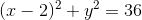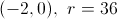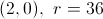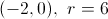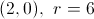A circle is defined by an equation in the format.
The center is indicated by the point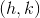and the radius.
In the equation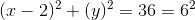, the center is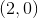and the radius is.